# Pagination of Database Query Results

We have a typical database base backed web application. It can be a rich client. It can be a NoSQL database. Whatever, but for the moment maybe a transactional SQL database and a web application will be used as the most common and best understood example.

We kind of construct a query, of which the result set is so large, that we do not want to transfer, render and display it all at once. Think of Google, where you might have millions of hits and only see the first 10 or 100 or so. Now you can navigate through the result set, usually by going to the next pages successively.

Nice web applications tell you how many records there are exactly. And then calculate how to split this up into a large number of pages and allowing you to go to an arbitrary page, or at least to some of the pages (last, next 10 pages) immediately.

There are several problems with this. First of all: the set you are basing this on changes with time. This could be fixed by creating some temporary snapshot in memory, in the database or in some kind of db-cache and keeping that around for some time. This is expensive and usually not worth the price, so we live with some misbehavior.

The second problem is the count. Count actually needs to do a full scan of the result set, there is no decent short cut. Now it would usually be good enough to estimate the size of the result set and that is exactly what google does. Sometimes the estimation is ridiculously bad, but we have gotten used to it. That is much better than using the real count, because it really speeds up the application by a great factor and improves user experience overall. Of course it is a bit more challenging to program an estimation and only worth it for big result sets. We could still jump to any of the next 10 pages by just increasing the size of the current query by 11 and showing the required segment. Since we sort the result by something, we could just get the first N results sorted backwards and then wrap that in a query that sorts them forward. So dropping the count can help for performance without too much loss in functionality.

Now the next problem is, that having navigated through a lot of pages, the usual way of doing a query and then constraining it with some mechanism like „rownum“, „row_number“, „limit/offset“ to the subset we want to display always requires obtaining the resultset from the beginning to our page. Usually that does not matter, because we navigate through the application manually and that means not too many pages from the beginning. But it can become an issue, if the application is heavily used.

Now we sort by something. Usually not the id, but some business logic relevant keys or a combination. They do not have to be unique, but we can always add the ID (or more generally the primary key) as the last sort key to make it unique. Then the next page can be found by just adding a condition based on our sort key. Assuming we want to show N records. The query might be
 SELECT * FROM TABX    WHERE cond1 AND cond2    ORDER BY A, B, C ASC    LIMIT n; 
Now the last record of the result has A=a1, B=b1, C=c1.
Then the next page can be obtained by
 SELECT * FROM TABX    WHERE cond1 AND cond2     AND (A>a1 OR A=a1 AND B>b1 OR A=a1 AND B=b1 AND C>c1)    ORDER BY A, B, C ASC    LIMIT n; 
To skip three pages and get the fourth of the following pages is done like this:
 SELECT * FROM TABX    WHERE cond1 AND cond2     AND (A>a1 OR A=a1 AND B>b1 OR A=a1 AND B=b1 AND C>c1)    ORDER BY A, B, C ASC   LIMIT n OFFSET 3*n; 
We do use (or simulate) OFFSET here, but in a more intelligent way, because we do not force it to work harder than necessary by rebasing our query on what we already know.

We get a bit more consistency, because new records being inserted in the beginning will not be seen, but they will at least not disturb the succession of our result set. Maybe that is worth more than the performance gain, because we get it with a reasonable effort. caches, snapshots or even keeping it in „session memory“ are not really serious approaches to this issue, they will just sooner or later blowup our application in production, at the worst possible moment.

# JSON instead of Java Serialization: The solution?

We start recognizing that Serialization is not such a good idea.

It is cool and can really work on a wide range of objects, even including complex and cyclic reference graphs. And it was essential for some older Java frameworks like EJB and RMI, which allowed remote access to Java objects and classes.

But it is no longer the future, Oracle will soon deprecate and later remove it. And it will happen this time, even though they really keep stuff around for a long time due to compatibility requirements.

Just to recap: it opens up security discussion, it opens up hidden behavior and makes it harder to reason about code, it creates tight coupling between remote components and it can result in bugs, that only occur at runtime and cannot be discovered at compile time. In short, it is not resilient.

So we need something else. Obvious candidates are XML, YAML and JSON. XML is of course an option and is powerful enough to do many things, but often a bit too clumbsy and too much boiler plate, so we try to move away from it. YAML and JSON kind of do the same thing, but it seems that JSON is winning the race and we all need to know JSON and many of us tend to skip YAML.

So why not use JSON. It is easy, it has good libraries and we can even find databases that work with JSON.

What JSON can express very well are scalars, lists and maps and combinations of these. This is quite exactly what we have in Perl, JavaScript or Clojure as basic building blocks. These languages support object oriented programming, but for simple stuff we go with these basic building blocks. And objects can be modelled as (hash-)maps, with the attribute names as keys. Actually JSON is valid JavaScript code.

We do have to change our thinking when moving from Java Serialization to JSON. JSON does not store any serializable object but just data. Maybe that is enough and that is what we actually want. It totally works in heterogeneous environments, where we are using different programming languages or different implementations.

There are good libraries. I have tried two, Jackson and GSON which both work well, recently mostly Jackson. It is important to think of Clojure, JavaScript, Perl or something like that without objects. So we loose type information, which can be considered good or bad, but if we can arrange ourselves with it, we avoid the tight coupling. JavaBeans are expressed exactly the same as a HashMap with the attribute names as keys. We can provide the top level class when deserializing, but at the child levels it will not be able to figure that out, if it relies on runtime information.

## Example Code

Here it has been tried out. Find full example code on github.

A class that contains all kinds of stuff. Not prepared for really putting in nulls, but it is just experimental code…

 package net.itsky.jackson;

 import java.util.List; import java.util.Map; import java.util.Set; public class TestObject {     private Long l;     private String s;     private Boolean b;     private Set set;     private List list;     private Map map;     public TestObject(Long l, String s, Boolean b, Set set, List list, Map map) {         this.l = l;         this.s = s;         this.b = b;         this.set = set;         this.list = list;         this.map = map;     }     public TestObject() {         // only for framework purposes     }     public Long getL() {         return l;     }     public String getS() {         return s;     }     public Boolean getB() {         return b;     }     public Set getSet() {         return set;     }     public List getList() {         return list;     }     public Map getMap() {         return map;     } 

    @Override     public String toString() {         return getClass().getSimpleName() + "(" +                "l=" + l + " (" + l.getClass() + ") "                 + " s=\"" + s + "\" (" + s.getClass() + ") "                 + " b=" + b + " (" + b.getClass() + ") "                 + " set=" + set  + " (" + set.getClass() + ") "                 + " list=" + list + " (" + list.getClass() + ") "                 + " map=" + map + " (" + map.getClass() + "))";     } } 
And this is used for running everything. To play around more, it should probably be moved to tests..
 package net.itsky.jackson;

 import com.fasterxml.jackson.databind.ObjectMapper; import com.fasterxml.jackson.databind.ObjectWriter; import com.google.common.collect.ImmutableList; import com.google.common.collect.ImmutableMap; import com.google.common.collect.ImmutableSet; import java.io.StringReader; import java.util.List; import java.util.Map; import java.util.Set; public class App { 

    public static void main(String[] args) {         try {             Set s1 = ImmutableSet.of(1, 2, 3);             Set s2 = ImmutableSet.of(1, 2, 3);             Map m1 = ImmutableMap.of("A", "abc", "B", 3L, "C", s1);             List l1 = ImmutableList.of("i", "e", "a", "o", "u");             TestObject t1 = new TestObject(30303L, "uv", true, s2, l1, m1);             Map m2 = ImmutableMap.of("r", "r101", "s", 202, "t", t1);             List l2 = ImmutableList.of("ä", "ö", "ü", "å", "ø");             Set s3 = ImmutableSet.of("x", "y", "z");             TestObject t2 = new TestObject(40404L, "ijk", false, s3, l2, m2);             ObjectMapper mapper = new ObjectMapper();             ObjectWriter writer = mapper.writerWithDefaultPrettyPrinter();             System.out.println("t2=" + t2);             String json = writer.writeValueAsString(t2);             System.out.println("json=" + json);             StringReader stringReader = new StringReader(json);             TestObject t3 = mapper.readValue(stringReader, TestObject.class);             System.out.println("t3=" + t3);         } catch (Exception ex) {             RuntimeException rex;             if (ex instanceof RuntimeException) {                 rex = (RuntimeException) ex;             } else {                 rex = new RuntimeException(ex);             }             throw rex;         }     } } 
And here is the output:
 t2=TestObject(l=40404 (class java.lang.Long)  s="ijk" (class java.lang.String)   b=false (class java.lang.Boolean)   set=[x, y, z] (class com.google.common.collect.RegularImmutableSet)   list=[ä, ö, ü, å, ø] (class com.google.common.collect.RegularImmutableList)   map={r=r101, s=202,  t=TestObject(l=30303 (class java.lang.Long)   s="uv" (class java.lang.String)  b=true (class java.lang.Boolean)   set=[1, 2, 3] (class com.google.common.collect.RegularImmutableSet)   list=[i, e, a, o, u] (class com.google.common.collect.RegularImmutableList)   map={A=abc, B=3, C=[1, 2, 3]} (class com.google.common.collect.RegularImmutableMap))}  (class com.google.common.collect.RegularImmutableMap)) json={   "l" : 40404,   "s" : "ijk",   "b" : false,   "set" : [ "x", "y", "z" ],   "list" : [ "ä", "ö", "ü", "å", "ø" ],   "map" : {     "r" : "r101",     "s" : 202,     "t" : {       "l" : 30303,       "s" : "uv",       "b" : true,       "set" : [ 1, 2, 3 ],       "list" : [ "i", "e", "a", "o", "u" ],       "map" : {         "A" : "abc",         "B" : 3,         "C" : [ 1, 2, 3 ]       }     }   } } t3=TestObject(l=40404 (class java.lang.Long)  s="ijk" (class java.lang.String)   b=false (class java.lang.Boolean)   set=[x, y, z] (class java.util.HashSet)   list=[ä, ö, ü, å, ø] (class java.util.ArrayList)   map={r=r101, s=202,  t={l=30303, s=uv, b=true,  set=[1, 2, 3],  list=[i, e, a, o, u],  map={A=abc, B=3, C=[1, 2, 3]}}}   (class java.util.LinkedHashMap))

 

Process finished with exit code 0 

So the immediate object and its immediate attributes were deserialized properly to what we provided. But everything inside went to maps, lists and scalars.

The intermediate JSON does not carry the type information at all, so this is the best that can be done.
Often it is useful what we want. If not, we need to find something else or see if we can tweak JSON to carry type information.

It will be interesting to explore other serialization protocols…

# Phone Numbers and E-Mail Addresses

Most data that we deal with are strings or numbers or booleans and combinations of these into classes and collections. Dates can be expressed as string or number, but have enough specific logic to be seen as a fourth group of data. All these have interesting aspects, some of which have been discussed in this blog already.

Now phone numbers are by an naïve approach numbers or strings, but very soon we see that they have their own specific aspects. The same applies for email addresses which can be represented as strings.

Often projects go by their own „simplified“ specification of what an email address or a phone number is, how to parse, compare and render them. In the end of the day the simplification is harder to tame than the real solution, because it needs to be maintained and specified by the project team rather than being based on a proven library. And once in a while „edge cases“ occur, that cannot be ignored and that make the „home grown“ library even more complex.

Behind phone numbers and email addresses there are well defined and established standards and they are hard to understand thoroughly within the constrained time budget of a typical „business project“, because the time should be allocated to enhancing the business logic and not to reinventing the basics. Unless there is a real need to do so, of course.

Just to give an idea: When phone numbers are parsed or provided by user input, they can start with a „+“ sign or use some country specific logic to express, to which country they belong. And then the „+1“, for example, does not stand for the United States alone, but also for Canada and some smaller countries that are in some way associated with the United States or Canada. Further analysis of the number is required to know about that. The prefix for international number is often „00“, but in the United States it is „011“ and there were and are some other variants, that are still frequently used. Some people like to write something like „+49(0)431 77 88 99 11 1“ instead of „+49 431 77 88 99 11 1“. We can constrain the input to the variants we happen to think of and force the supplier of data to comply, but why bother? Why not accept legitimate formats, as long as they are correct and unambiguous?

Now for E-Mail-addresses there is the famous one page regular expression to recognize correct email addresses which is even by itself not totally complete. Find it at the bottom of the article…

Of course it includes some rarely used variants of email addresses that were once used and have not been completely abolished officially, but it is hard to draw and exact border for this.

So the general recommendation is to find a good library for working with email addresses and phone numbers. Maybe the library can even to some extent eliminate input strings that are formally complying the format, but know to be incorrect by knowing about numbering schemes world wide or about email domains or even by performing lookups.

Another strong recommendation is to store data like email addresses and phone numbers in a technical format, that is in the example of phone numbers always starting with a „+“ followed by digits only. For input any positioning of spaces is accepted, for output the library knows how to format it correctly. This allows selecting by the numbers without dealing with complex formatting, by just using the technical format in the query as well.

For Java (and thus for many JVM-languages), C++ and JavaScript there is an excellent library from Google for dealing with phone numbers. For E-Mails something like apache commons email validator is a way to go.

Keep in mind that for E-Mail addresses and phone numbers, the ultimate way of verification is to send them a link or a code that they need to enter. In the end of the day it is insufficient to rely only on formal verification without this final step.

But still issues remain for transforming data into a canonical technical format for storing them, formatting data for display etc. And there is a huge added value, if we can reliably recognize formally false entries early, when the user can still easily react to it, rather than waiting for an email/SMS/phone call being processed, which may fail when the user is no longer on our „registration site“. And we can process data which has already been verified by a third party, but still we want to parse it to recognize obvious errors.

The concrete libraries may be outdated by the time you are reading this, or they may not be applicable for the language environment that you are using, but please make an effort to find something similar.

So, please use good libraries, that are like to be found for the environment that you are using and write yourself what creates value for your project or organization. Unless your goal is really to write a better library. Better invest the time into areas where there are still no good libraries around.

And as always, you may understand email addresses and phone numbers as an example for a more general idea.

## E-Mail Regex

(?:(?:\r\n)?[ \t])*(?:(?:(?:[^()<>@,;:\\". \000-\031]+(?:(?:(?:\r\n)?[ \t] )+|\Z|(?=[$"()<>@,;:\\".\[$]))|"(?:[^\"\r\\]|\\.|(?:(?:\r\n)?[ \t]))*"(?:(?: \r\n)?[ \t])*)(?:\.(?:(?:\r\n)?[ \t])*(?:[^()<>@,;:\\". \000-\031]+(?:(?:( ?:\r\n)?[ \t])+|\Z|(?=[$"()<>@,;:\\".\[$]))|"(?:[^\"\r\\]|\\.|(?:(?:\r\n)?[ \t]))*"(?:(?:\r\n)?[ \t])*))*@(?:(?:\r\n)?[ \t])*(?:[^()<>@,;:\\". \000-\0 31]+(?:(?:(?:\r\n)?[ \t])+|\Z|(?=[$"()<>@,;:\\".\[$]))|$([^\[$\r\\]|\\.)*\ ](?:(?:\r\n)?[ \t])*)(?:\.(?:(?:\r\n)?[ \t])*(?:[^()<>@,;:\\". \000-\031]+ (?:(?:(?:\r\n)?[ \t])+|\Z|(?=[$"()<>@,;:\\".\[$]))|$([^\[$\r\\]|\\.)*\](?: (?:\r\n)?[ \t])*))*|(?:[^()<>@,;:\\". \000-\031]+(?:(?:(?:\r\n)?[ \t])+|\Z |(?=[$"()<>@,;:\\".\[$]))|"(?:[^\"\r\\]|\\.|(?:(?:\r\n)?[ \t]))*"(?:(?:\r\n) ?[ \t])*)*\<(?:(?:\r\n)?[ \t])*(?:@(?:[^()<>@,;:\\". \000-\031]+(?:(?:(?:\ r\n)?[ \t])+|\Z|(?=[$"()<>@,;:\\".\[$]))|$([^\[$\r\\]|\\.)*\](?:(?:\r\n)?[ \t])*)(?:\.(?:(?:\r\n)?[ \t])*(?:[^()<>@,;:\\". \000-\031]+(?:(?:(?:\r\n) ?[ \t])+|\Z|(?=[$"()<>@,;:\\".\[$]))|$([^\[$\r\\]|\\.)*\](?:(?:\r\n)?[ \t] )*))*(?:,@(?:(?:\r\n)?[ \t])*(?:[^()<>@,;:\\". \000-\031]+(?:(?:(?:\r\n)?[ \t])+|\Z|(?=[$"()<>@,;:\\".\[$]))|$([^\[$\r\\]|\\.)*\](?:(?:\r\n)?[ \t])* )(?:\.(?:(?:\r\n)?[ \t])*(?:[^()<>@,;:\\". \000-\031]+(?:(?:(?:\r\n)?[ \t] )+|\Z|(?=[$"()<>@,;:\\".\[$]))|$([^\[$\r\\]|\\.)*\](?:(?:\r\n)?[ \t])*))*) *:(?:(?:\r\n)?[ \t])*)?(?:[^()<>@,;:\\". \000-\031]+(?:(?:(?:\r\n)?[ \t])+ |\Z|(?=[$"()<>@,;:\\".\[$]))|"(?:[^\"\r\\]|\\.|(?:(?:\r\n)?[ \t]))*"(?:(?:\r \n)?[ \t])*)(?:\.(?:(?:\r\n)?[ \t])*(?:[^()<>@,;:\\". \000-\031]+(?:(?:(?: \r\n)?[ \t])+|\Z|(?=[$"()<>@,;:\\".\[$]))|"(?:[^\"\r\\]|\\.|(?:(?:\r\n)?[ \t ]))*"(?:(?:\r\n)?[ \t])*))*@(?:(?:\r\n)?[ \t])*(?:[^()<>@,;:\\". \000-\031 ]+(?:(?:(?:\r\n)?[ \t])+|\Z|(?=[$"()<>@,;:\\".\[$]))|$([^\[$\r\\]|\\.)*\]( ?:(?:\r\n)?[ \t])*)(?:\.(?:(?:\r\n)?[ \t])*(?:[^()<>@,;:\\". \000-\031]+(? :(?:(?:\r\n)?[ \t])+|\Z|(?=[$"()<>@,;:\\".\[$]))|$([^\[$\r\\]|\\.)*\](?:(? :\r\n)?[ \t])*))*\>(?:(?:\r\n)?[ \t])*)|(?:[^()<>@,;:\\". \000-\031]+(?:(? :(?:\r\n)?[ \t])+|\Z|(?=[$"()<>@,;:\\".\[$]))|"(?:[^\"\r\\]|\\.|(?:(?:\r\n)? [ \t]))*"(?:(?:\r\n)?[ \t])*)*:(?:(?:\r\n)?[ \t])*(?:(?:(?:[^()<>@,;:\\". \000-\031]+(?:(?:(?:\r\n)?[ \t])+|\Z|(?=[$"()<>@,;:\\".\[$]))|"(?:[^\"\r\\]| \\.|(?:(?:\r\n)?[ \t]))*"(?:(?:\r\n)?[ \t])*)(?:\.(?:(?:\r\n)?[ \t])*(?:[^()<> @,;:\\". \000-\031]+(?:(?:(?:\r\n)?[ \t])+|\Z|(?=[$"()<>@,;:\\".\[$]))|" (?:[^\"\r\\]|\\.|(?:(?:\r\n)?[ \t]))*"(?:(?:\r\n)?[ \t])*))*@(?:(?:\r\n)?[ \t] )*(?:[^()<>@,;:\\". \000-\031]+(?:(?:(?:\r\n)?[ \t])+|\Z|(?=[$"()<>@,;:\\ ".\[$]))|$([^\[$\r\\]|\\.)*\](?:(?:\r\n)?[ \t])*)(?:\.(?:(?:\r\n)?[ \t])*(? :[^()<>@,;:\\". \000-\031]+(?:(?:(?:\r\n)?[ \t])+|\Z|(?=[$"()<>@,;:\\".\[$]))|$([^\[$\r\\]|\\.)*\](?:(?:\r\n)?[ \t])*))*|(?:[^()<>@,;:\\". \000- \031]+(?:(?:(?:\r\n)?[ \t])+|\Z|(?=[$"()<>@,;:\\".\[$]))|"(?:[^\"\r\\]|\\.|( ?:(?:\r\n)?[ \t]))*"(?:(?:\r\n)?[ \t])*)*\<(?:(?:\r\n)?[ \t])*(?:@(?:[^()<>@,; :\\". \000-\031]+(?:(?:(?:\r\n)?[ \t])+|\Z|(?=[$"()<>@,;:\\".\[$]))|$([ ^\[$\r\\]|\\.)*\](?:(?:\r\n)?[ \t])*)(?:\.(?:(?:\r\n)?[ \t])*(?:[^()<>@,;:\\" . \000-\031]+(?:(?:(?:\r\n)?[ \t])+|\Z|(?=[$"()<>@,;:\\".\[$]))|$([^\[\ ]\r\$|\\.)*\](?:(?:\r\n)?[ \t])*))*(?:,@(?:(?:\r\n)?[ \t])*(?:[^()<>@,;:\\".\ [\] \000-\031]+(?:(?:(?:\r\n)?[ \t])+|\Z|(?=[$"()<>@,;:\\".\[$]))|$([^\[$\ r\\]|\\.)*\](?:(?:\r\n)?[ \t])*)(?:\.(?:(?:\r\n)?[ \t])*(?:[^()<>@,;:\\". \000-\031]+(?:(?:(?:\r\n)?[ \t])+|\Z|(?=[$"()<>@,;:\\".\[$]))|$([^\[$\r\\] |\\.)*\](?:(?:\r\n)?[ \t])*))*)*:(?:(?:\r\n)?[ \t])*)?(?:[^()<>@,;:\\". \0 00-\031]+(?:(?:(?:\r\n)?[ \t])+|\Z|(?=[$"()<>@,;:\\".\[$]))|"(?:[^\"\r\\]|\\ .|(?:(?:\r\n)?[ \t]))*"(?:(?:\r\n)?[ \t])*)(?:\.(?:(?:\r\n)?[ \t])*(?:[^()<>@, ;:\\". \000-\031]+(?:(?:(?:\r\n)?[ \t])+|\Z|(?=[$"()<>@,;:\\".\[$]))|"(? :[^\"\r\\]|\\.|(?:(?:\r\n)?[ \t]))*"(?:(?:\r\n)?[ \t])*))*@(?:(?:\r\n)?[ \t])* (?:[^()<>@,;:\\". \000-\031]+(?:(?:(?:\r\n)?[ \t])+|\Z|(?=[$"()<>@,;:\\". \[$]))|$([^\[$\r\\]|\\.)*\](?:(?:\r\n)?[ \t])*)(?:\.(?:(?:\r\n)?[ \t])*(?:[ ^()<>@,;:\\". \000-\031]+(?:(?:(?:\r\n)?[ \t])+|\Z|(?=[$"()<>@,;:\\".\[$ ]))|$([^\[$\r\\]|\\.)*\](?:(?:\r\n)?[ \t])*))*\>(?:(?:\r\n)?[ \t])*)(?:,\s*( ?:(?:[^()<>@,;:\\". \000-\031]+(?:(?:(?:\r\n)?[ \t])+|\Z|(?=[$"()<>@,;:\\ ".\[$]))|"(?:[^\"\r\\]|\\.|(?:(?:\r\n)?[ \t]))*"(?:(?:\r\n)?[ \t])*)(?:\.(?:( ?:\r\n)?[ \t])*(?:[^()<>@,;:\\". \000-\031]+(?:(?:(?:\r\n)?[ \t])+|\Z|(?=[ $"()<>@,;:\\".\[$]))|"(?:[^\"\r\\]|\\.|(?:(?:\r\n)?[ \t]))*"(?:(?:\r\n)?[ \t ])*))*@(?:(?:\r\n)?[ \t])*(?:[^()<>@,;:\\". \000-\031]+(?:(?:(?:\r\n)?[ \t ])+|\Z|(?=[$"()<>@,;:\\".\[$]))|$([^\[$\r\\]|\\.)*\](?:(?:\r\n)?[ \t])*)(? :\.(?:(?:\r\n)?[ \t])*(?:[^()<>@,;:\\". \000-\031]+(?:(?:(?:\r\n)?[ \t])+| \Z|(?=[$"()<>@,;:\\".\[$]))|$([^\[$\r\\]|\\.)*\](?:(?:\r\n)?[ \t])*))*|(?: [^()<>@,;:\\". \000-\031]+(?:(?:(?:\r\n)?[ \t])+|\Z|(?=[$"()<>@,;:\\".\[\ ]]))|"(?:[^\"\r\$|\\.|(?:(?:\r\n)?[ \t]))*"(?:(?:\r\n)?[ \t])*)*\<(?:(?:\r\n) ?[ \t])*(?:@(?:[^()<>@,;:\\". \000-\031]+(?:(?:(?:\r\n)?[ \t])+|\Z|(?=[$" ()<>@,;:\\".\[$]))|$([^\[$\r\\]|\\.)*\](?:(?:\r\n)?[ \t])*)(?:\.(?:(?:\r\n) ?[ \t])*(?:[^()<>@,;:\\". \000-\031]+(?:(?:(?:\r\n)?[ \t])+|\Z|(?=[$"()<> @,;:\\".\[$]))|$([^\[$\r\\]|\\.)*\](?:(?:\r\n)?[ \t])*))*(?:,@(?:(?:\r\n)?[ \t])*(?:[^()<>@,;:\\". \000-\031]+(?:(?:(?:\r\n)?[ \t])+|\Z|(?=[$"()<>@, ;:\\".\[$]))|$([^\[$\r\\]|\\.)*\](?:(?:\r\n)?[ \t])*)(?:\.(?:(?:\r\n)?[ \t] )*(?:[^()<>@,;:\\". \000-\031]+(?:(?:(?:\r\n)?[ \t])+|\Z|(?=[$"()<>@,;:\\ ".\[$]))|$([^\[$\r\\]|\\.)*\](?:(?:\r\n)?[ \t])*))*)*:(?:(?:\r\n)?[ \t])*)? (?:[^()<>@,;:\\". \000-\031]+(?:(?:(?:\r\n)?[ \t])+|\Z|(?=[$"()<>@,;:\\". \[$]))|"(?:[^\"\r\\]|\\.|(?:(?:\r\n)?[ \t]))*"(?:(?:\r\n)?[ \t])*)(?:\.(?:(?: \r\n)?[ \t])*(?:[^()<>@,;:\\". \000-\031]+(?:(?:(?:\r\n)?[ \t])+|\Z|(?=[$"()<>@,;:\\".\[$]))|"(?:[^\"\r\\]|\\.|(?:(?:\r\n)?[ \t]))*"(?:(?:\r\n)?[ \t]) *))*@(?:(?:\r\n)?[ \t])*(?:[^()<>@,;:\\". \000-\031]+(?:(?:(?:\r\n)?[ \t]) +|\Z|(?=[$"()<>@,;:\\".\[$]))|$([^\[$\r\\]|\\.)*\](?:(?:\r\n)?[ \t])*)(?:\ .(?:(?:\r\n)?[ \t])*(?:[^()<>@,;:\\". \000-\031]+(?:(?:(?:\r\n)?[ \t])+|\Z |(?=[$"()<>@,;:\\".\[$]))|$([^\[$\r\\]|\\.)*\](?:(?:\r\n)?[ \t])*))*\>(?:( ?:\r\n)?[ \t])*))*)?;\s*)

# Ranges of Dates and Times

In Software we often deal with ranges of dates and times.

Let us look at it from the perspective of an end user.

When we say something like „from 2020-03-07 to 2019-03-10“ we mean the set of all timestampssuch that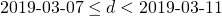or more accurately: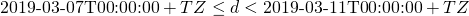Important is, that we mean to include the whole 24 hour day of 2019-03-10. Btw. please try to get used to the ISO-date even when writing normal human readable texts, it just makes sense…

Now when we are not talking about dates, but about times or instants of time, the interpretation is different.
When we say sonmething like „from 07:00 to 10:00“ or „from 2020-03-10T07:00:00+TZ to 2020-04-11T09:00:00+TZ“, we actually mean the set of all timestampssuch thator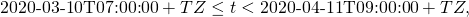respectively. It is important that we have to add one in case of date only (accuracy to one day) and we do not in case of finer grained date/time information. The question if the upper bound is included or not is not so important in our everyday life, but it proves that commonly the most useful way is not to include the upper bound. If you prefer to have all options, it is a better idea to employ an interval library, i.e. to find one or to write one. But for most cases it is enough to exclude the upper limit. This guarantees disjoint adjacent intervals which is usually what we want. I have seen people write code that adds 23:59:59.999 to a date and compares with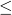instead of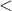, but this is an ugly hack that needs a lot of boiler plate code and a lot of time to understand. Use the exclusive upper limit, because we have it.

Now the requirement is to add one day to the upper limit to get from the human readable form of date-only ranges to something computers can work with. It is a good thing to agree on where this transformation is made. And to do it in such a way that it even behaves correctly on those dates where daylight saving starts or ends, because adding one day might actually mean „23 hours“ or „25 hours“. If we need to be really very accurate, sometimes switch seconds need to be added.

Just another issue has come up here. Local time is much harder than UTC. We need to work with local time on all kinds of user interfaces for humans, with very few exceptions like for pilots, who actually work with UTC. But local date and time is ambiguous for one hour every year and at least a bit special to handle for these two days where daylight saving starts and ends. Convert dates to UTC and work with that internally. And convert them to local date on all kinds of user interfaces, where it makes sense, including documents that are printed or provided as PDFs, for example. When we work with dates without time, we need to add one day to the upper limit and then round it to the nearest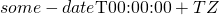for our timezone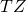or know when to add 23, 24 or 25 hours, respectively, which we do not want to know, but we need to use modern time libraries like the java.time.XXX stuff in Java, for example.

Working with date and time is hard. It is important to avoid making it harder than it needs to be. Here some recommendations:

• Try to use UTC for the internal use of the software as much as possible
• Use local date or time or date and time in all kinds of user interfaces (with few exceptions)
• add one day to the upper limit and round it to the nearest midnight of local time exactly once in the stack
• exclude the upper limit in date ranges
• Use ISO-date formats even in the user interfaces, if possible

# UUIDs revisited

UUIDs have proven useful in many circumstances.
We have basically two main variants:

• The UUID is calculated as a combination of the Ethernet-MAC-address, the timestamp and a counter.
• The UUID is calculated using a good random number generator

While variant 1 provides for a good uniqueness, there are some issues with it. Today we use mostly virtualized servers, which means that the MAC-address is coming from a configuration file and no longer guaranteed to be world wide unique. And we give away some information with the UUID, that we do not necessarily want to give away.

Variant 2 can be proven to have an acceptably low risk of collisions, but this is only true when using really good random number generators, which cannot always be guaranteed. Also it introduces an uncertainty in an area where we do not need it. We need to worry about this uniqueness, at least a little but, which is unnecessary.

So the question is, if we can rethink variant 1.

Assuming, that our software runs on our server farm. There may be a few hundred or thousand or even millions of virtual or physical servers. Now the organization does have a way to uniquely identify their servers. Of course we only need to consider the servers that are relevant for the application. Maybe an ID for the service instance instead of the server is even better. We may assume a numerical ID or something or have a table to map IP addresses and the like to such an ID. Some thinking is still required on how to do this. We can fill the digits that we do not need with random numbers.

The next problem, that timestamps can be abused to find out something that should not be found out could be resolved by running the timestamp part or even the ID part (including a random number) through a symmetric encryption or simply some bijective function that is kept as a secret.

In many circumstances there is nothing wrong with customizing the UUID-generation to some „local“ standard, if this is well understood and carefully implemented.

# Flashsort in Scala

There is now also an implementation of Flashsort in Scala.

In order to solve the requirement of sorting part of an array that is needed as part of flashsort, an heapsort implementation in Scala that can be constrained to a part of an array has been included as well. Heapsort was chosen, because it can sort in place and it has a guaranteed performance of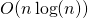. Mergesort or quicksort would have been reasonable choices as well. Some implentations even use insertion sort for this step, because the sections are small.

# Flashsort in Ruby

Deutsch

There is a simple implementation of Flashsort in Ruby, after having already provided an implementation in C. The C-implementation is typically faster than the libc-function qsort, but this depends always on the data and on how well the metric-function has been written, that is needed on top of the comparison function for Flashsort. You can think of this metric function as some kind of monotonic hash function. So we have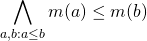This additionally needed function of method is not really there, apart from numerical values, so we really have to invest some time into writing it. This makes the use of Flashsort a bit harder. A good metric function is crucial for good performance, but for typical text files quite trivial implentations already outperform classical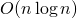algorithms like Heapsort and Quicksort and Mergesort for larger amounts of data.

This blog article shows other sorting algorithms for Ruby.

# Indexing of Arrays and Lists

We index arrays with integers. Lists also, at least the ones that allow random access. And sizes of collections are also integers.
This allows forentries in Java and typical JVM languages, because integers are actually considered to be 32bit. Actually we could think of one more entry, using indices, but then we would not be able to express the size in an signed integer. This stuff is quite deeply built into the language, so it is not so easy to break out of this. And 2’000’000’000 entries are a lot and take a lot of time to process. At least it was a lot in the first few years of Java. There should have been an unsigned variant of integers, which would in this case allow for 4’000’000’000 entries, when indexing by an uint32, but that would not really solve this problem. C uses 64 bit integers for indexing of arrays on 64 bit systems.

It turns out that we would like to be able to index arrays using long instead of int. Now changing the java arrays in a way that they could be indexed by long instead of int would break a lot of compatibility. I think this is impossible, because Java claims to retain very good backward compatibility and this reliability of both the language and the JVM has been a major advantage. Now a second type of arrays, indexed by long, could be added. This would imply even more complexity for APIs like reflection, that have to deal with all cases for parameters, where it already hurts that the primitives are no objects and arrays are such half-objects. So it will be interesting, what we can find in this area in the future.

For practical use it is a bit easier. We can already be quite happy with a second set of collections, let them be called BigCollections, that have sizes that can only be expressed with long and that are indexed in cases where applicable with longs. Now it is not too hard to program a BigList by internally using an array of arrays or an array of arrays of arrays and doing some arithmetic to calculate the internal indices from the long (int64) index given in the API. Actually we can buy some performance gain when resizing happens, because this structure, if well done, allows for more efficient resizing. Based on this all kinds of big collections could be built.

# Intervals

Intervals are subsets of a universe, that are defined by upper and lower boundaries. Typically we think about real numbers, but any totally ordered universe allows the definition of intervals.

Intervals are defined by lower and upper boundaries, which can be a limiting number or unlimited, typically written as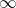for the upper bound andfor the lower bound. The boundaries can be included or excluded. So the following combinations exist for a universe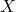: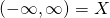unlimited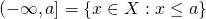half open, lower unlimited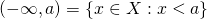open, lower unlimited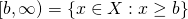half open, upper unlimited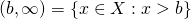open, upper unlimited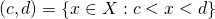openhalf open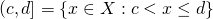half open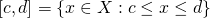closedit is sometimes useful to consider the empty set as an interval as well

The words „open“ and „closed“ refer to our usual topology of real numbers, but they do not necessarily retain their topological meaning when we extend the concept to our typical data types.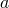,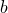,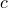andin the notation above do not have to be members of, as long as the comparison is defined between them and all members of. So we could for example meaningfully define for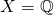the interval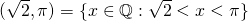.

As soon as we do not imply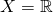we always have to make this clear… And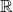is kind of hard to really work with in software on computers with physically limited memory and CPU power.

Intervals have some relevance in software systems.

We sometimes have a business logic that actually relies on them and instead programming somehow around it, it is clearer and cleaner to actually work with intervals. For example, we can have a public transport scheduling system and we deal with certain time intervals in which different schedules apply than during the rest of the day. Or we have a system that records downtimes of servers and services and these are quite naturally expressed as intervals of some date-time datatype. It is usually healthy to consider all the cases mentioned above rather than ignoring the question if the boundary with probability zero of actually happening or having ugly interval limits like 22:59:59.999.

The other case is interval arithmetic. This means, we do floating point calculations by taking into account that we have an inaccuracy. So instead of numbers we have intervals. When we add two intervals, we get. In the same way we can multiply and subtract and even divide, as long as we can stay clear of zero in the denominator. Or more generally we can define.
It does of course require some mathematical thinking to understand, if the result is an interval again or at least something we can deal with reasonably. Actually we are usually happy with replacing the result by an interval that is possibly a superset of the real result, ideally the minimal superset that can be expressed with our boundary type.

At this point we will probably discover a desire to expand the concept of intervals in a meaningful way to complex numbers. We can do this by working with open disks likeor closed disks like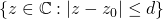. Or with rectangles based on two intervalsand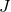like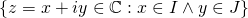.

These two areas are quite interesting and sometimes useful. Libraries have been written for both of them.

Often we discover, that intervals alone are not quite enough. We would like to do set operations with intervals, that is

union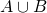intersection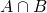set difference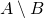While the intersection works just fine, as long as we include the empty setas an interval, unions and differences lead us to non-intervals. It turns out that interval-unions, sets that can be expressed as a union of a finite number of intervals, turn out to be a useful generalization, that is actually what we want to work with rather than with intervals. In this case we can drop the empty set as interval and just express it as the union of zero intervals.

There are some questions coming up, that are interesting to deal with:

normalization
Can we normalize interval-unions to some canonical form that allows safe and relyable comparison for equality?
is our universeactually discrete, so we can express all unlimited boundaries with closed boundaries?
interval lengths
Do we have a meaningful and useful way to measure the length of an interval or the total length of an interval-union, as long as they are limited? Or even for unlimited intervals?
collection interfaces
Do we want to implement a Set-interface in languages that have sets and an understanding of sets that would fit for intervals
implementation
How can we implement this ourselves?
implementation
Can we find useful implementations?

Having written a java library to support interval-unions on arbitrary Comparable types once in a project and having heard a speech about an interval library in Scala that ended up in using interval-unions in a pretty equivalent way, it might be interesting to write in the future about how to do this or what can be found in different languages to support us. For interval arithmetic some work has been done to create extensions or libraries for C and Fortran, that support this, while I was a student. So this is pretty old stuff and interesting mostly for the concepts, even if we are not going to move to Fortran because of this.

If there is interest I will write more about actual implementations and issues to address when using or writing them.